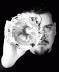## Stupid benchmarks and a bit of confusion

Please note: the following was done as an exercise in intellectual curiosity and not in any way an example of a real optimization. Any comments about "premature optimization" will be downvoted as soon as we get a voting system ;)

We're deep in the heart of micro-optimizing some extremely performance-intensive code when I stumbled across this:

``````if ( \$number == -1 ) {
# do something
}
``````

Clearly a numeric comparison isn't expensive and I managed to find a few areas where we could improve some performance, but out of curiosity, I decided to benchmark `\$number == -1`. The -1 is returned if a function failed (because throwing an exception would be far too expensive here) and we test for that. In reality, we only care if the number is less than 0. I was mildly curious to know if I could get a tiny performance increase in bit comparison (again, this was curiosity only. If I have to get this deep in optimization, I have more serious issues than this).

Perl, of course, offers a wide array of bit fiddling operators and they're often faster, though more obscure, than the "normal" way of doing things, though there are caveats if you start getting to "large" numbers (those values likely to overflow MAX_INT and friends). For example, a "fast" way to multiply a number by two is:

``````my \$double = \$num << 1;
``````

And a quick benchmark, using a variable to avoid constant folding (and verified using B::Deparse):

``````use Benchmark;

my \$num = 10;
timethese(
20_000_000,
{   'bits' => sub { \$num << 1 },
'mult' => sub { \$num * 2 },
}
);
``````

And that outputs:

``````Benchmark: timing 20000000 iterations of bits, mult...
bits:  2 wallclock secs ( 0.81 usr +  0.00 sys =  0.81 CPU) @ 24691358.02/s (n=20000000)
mult:  2 wallclock secs ( 1.06 usr +  0.00 sys =  1.06 CPU) @ 18867924.53/s (n=20000000)
``````

So you can see that sometimes you get a quick win from bit fiddling. I was using this in a prime number generator, but quickly blew past the `MAX_INT` size on my system (though it was very fast before I hit 2**64), so there are major caveats.

I have a 64 bit machine, so to test if a number is negative, I can using a bitwise `and` (&) against `1 << 63` (again, for very large numbers, this is dangerous!). Since we return -1, I compared against that, but since I can check for any negative number, I used a variety of strategies and came up with the following:

``````#!/usr/bin/env perl

use 5.12.0;
use Benchmark;

use constant IS_NEGATIVE => ( 1 << 63 );
my \$negative = -1;
my \$positive = 1;
timethese(
10_000_000,
{   'bitwise' => sub { \$negative & IS_NEGATIVE; \$positive & IS_NEGATIVE },
'== -1'   => sub { \$negative == -1;      \$positive == -1 },
'-1 =='   => sub { -1 == \$negative;      -1 == \$positive },
'<='      => sub { -1 <= \$negative;      -1 >= \$positive },
}
);
``````

And I received very consistent numbers across multiple runs:

``````Benchmark: timing 10000000 iterations of -1 ==, <=, == -1, bitwise...
-1 ==:  0 wallclock secs ( 0.49 usr +  0.00 sys =  0.49 CPU) @ 20408163.27/s (n=10000000)
<=:  1 wallclock secs ( 0.54 usr +  0.00 sys =  0.54 CPU) @ 18518518.52/s (n=10000000)
== -1:  1 wallclock secs ( 0.64 usr +  0.00 sys =  0.64 CPU) @ 15625000.00/s (n=10000000)
bitwise:  1 wallclock secs ( 0.69 usr +  0.00 sys =  0.69 CPU) @ 14492753.62/s (n=10000000)
``````

For this silly benchmark, I was surprised that the bitwise operator was so slow, but I was more surprised that `-1 == \$some_var` was so much faster than `\$some_var == -1`. So I wrote the following:

``````use 5.12.0;

use constant NEGATIVE => ( 1 << 63 );
my \$negative = -1;
sub bitwise { \$negative & NEGATIVE }
sub comp    { -1 == \$negative }
say bitwise;
say comp;
``````

And a quick `perl -MO=Deparse comp.pl` produces this:

``````LISTOP (0xa2ea60) leave 
OP (0xa32460) enter
COP (0xa31de0) nextstate
BINOP (0x9aa200) sassign
SVOP (0xa417b0) const  IV (0xa2c7a0) -1
COP (0xa2e410) nextstate
LISTOP (0xa2e8b0) say
OP (0xa366e0) pushmark
UNOP (0xa2e870) entersub 
UNOP (0xa3c250) null 
OP (0xa40d30) pushmark
UNOP (0xa2e830) null 
SVOP (0xa2e2c0) gv  GV (0x9f06e0) *bitwise
COP (0xa3be00) nextstate
LISTOP (0xa3bdc0) say
OP (0x8bc430) pushmark
UNOP (0xa2e530) entersub 
UNOP (0xa2e470) null 
OP (0xa36710) pushmark
UNOP (0xa2e4f0) null 
SVOP (0xa2e4b0) gv  GV (0x9f08f0) *comp
comp.pl syntax OK
``````

So ... the op trees look the same there. What am I misunderstanding?

I think the problem is that you're just printing details for the main program, not the subroutines.

```\$ perl -MO=Concise,bitwise,comp comp.pl
main::bitwise:
5   leavesub[1 ref] K/REFC,1 ->(end)
-      lineseq KP ->5
1         nextstate(main 294 up:5) v:*,&,\$,134219776 ->2
4         bit_and[t2] sK ->5
3            const(UV 9223372036854775808) s* ->4
main::comp:
a   leavesub[1 ref] K/REFC,1 ->(end)
-      lineseq KP ->a
6         nextstate(main 295 up:6) v:*,&,\$,134219776 ->7
9         eq sK/2 ->a
7            const(IV -1) s ->8Freelance Perl/Testing/Agile consultant and trainer. See http://www.allaroundtheworld.fr/ for our services. If you have a problem with Perl, we will solve it for you. And don't forget to buy my book! http://www.amazon.com/Beginning-Perl-Curtis-Poe/dp/1118013840/